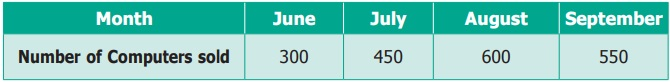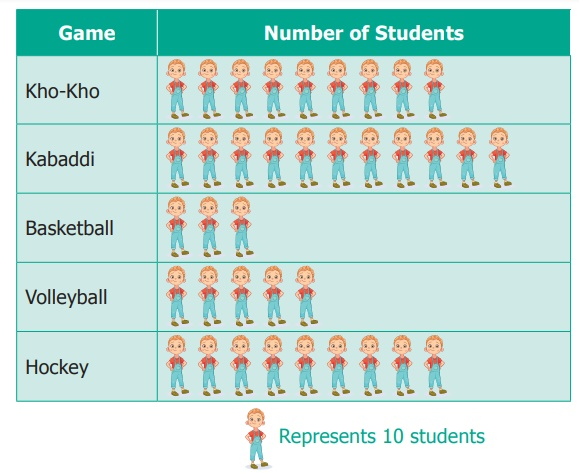Home | | Maths 6th Std | Exercise 5.2

# Exercise 5.2

6th Maths : Term 1 Unit 5 : Statistics : Exercise 5.2 : Text Book Back Exercises Questions with Answers, Solution

Exercise 5.2

1. Fill in the blanks.

(i) Ifrepresents 100 balls thenrepresents __________ balls. Answer: 150

(ii) If 200 is represented bythen 600 is represented by __________. Answer:(iii) Representation of data by using pictures is known as __________. Answer: pictograph

2. Draw a pictograph for the given data.3. The following table shows the number of tourists who visited the places in the month of May. Draw a pictograph.4. The following Pictograph shows the number of students playing different games in a school.(i) Which is the most popular game among the students?

(ii) Find the number of students playing Kabaddi.

(iii) Which two games are played by equal number of students?

(iv) What is the difference between the number of students playing Kho-Kho and Hockey?

(v) Which is the least popular game among the students?

Objective Type Questions

5. The pictorial representation for a phrase is a __________.

(a) Picto

(b) Tally mark

(c) Frequency

(d) Data

6. The representation of ‘one picture to many objects’ in a Pictograph is called __________.

(a) Tally mark

(b) Pictoword

(c) Scaling

(d) Frequency

7. A Pictograph is also known as __________.

(a) Pictoword

(b) Pictogram

(c) Pictophrase

(d) Pictograft

Exercise 5.2

1) i) 150

ii)iii) Pictograph

ii) 110

iii) Kho-Kho and Hockey

iv) 0

5) (a) Picto

6) (c) Scaling

7) (b) Pictogram

Tags : Questions with Answers, Solution | Statistics | Term 1 Chapter 5 | 6th Maths , 6th Maths : Term 1 Unit 5 : Statistics
Study Material, Lecturing Notes, Assignment, Reference, Wiki description explanation, brief detail
6th Maths : Term 1 Unit 5 : Statistics : Exercise 5.2 | Questions with Answers, Solution | Statistics | Term 1 Chapter 5 | 6th Maths